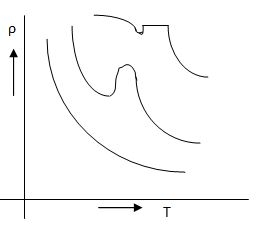# Resistivity of Semiconductor

The resistivity of semi conductor mostly depends on temperature and hence classifying a material as semi conductor basing on resistivity alone will not be correct. At very low temperatures near to the liquid helium the resistivity of the semi conductor may be equal to that of insulator. Therefore a better way is to consider the criterion from the graph given in Figure which is drawn for resistivity verses temperature for three typical semi conductor material. In all these cases the semiconductor exhibits a negative temperature co-efficient of resistivity atleast over a part of the temperature range. Since the resistivity is a function of temperature co-efficient of resistivity it can be written as

α1 = dρ/dT

For semi conductor the value is negative where

ρ is the resistivity of the material
T is Temperature
α1 – temperature co-efficient of resistivityResistivity vs Temperature
The valence electrons are not attached to individual atoms but are free to move in all directions among the atoms. These electrons are caIled conduction electrons and form 'free electron cloud' or 'free -electron gas' or the 'fermi gas'. When external field is applied to the metal, the free electron motion becomes directed. This type of motion is known a 'drift'.  The drift velocity is given by,
v = μe E

where

v - electron drift velocity
μe = electron mobility
E = applied electrical field.

The electric current flowing in any conductor is given by the amount of charge which flows in one second across any place of the conductor. The total number of electrons which cross any plane of cross section A in one second is equal to n (v x A) where, n is the free electrons per unit volume of the conductor i.e., electron density.

Charge carried by the electrons

I = e n v A
But v = μe A
Therefore, I = e n A μe E
The applied field E = v/l
Therefore, I = e n A μe v/l
But, R = ρl/A
R = v/I = l/A[1/ ne μe]
Therefore ρ = (1/ ne μe) Ω-m
and Conductivity σ = 1/ ρ = ne μe mho/m

Therefore in a semi conductor, charged carriers are both holes as well as electrons whereas in metals electrons are the only charged carriers.

Factors Affecting Resistivity of Semi Conductors

The resistivity of semi conductor are affected by the following factors :

(a) Impurities : The behaviour of a semiconductor mostly depends on the degree and type of impurity in crystal. Increase in conductivity is smaller for low values of impurities as compared to intrinsic type of semiconductor. For high values of impurity it behaves as an intrinsic semi conductor. Figure shows the curves on semi log paper showing the resistivities of semiconductor versus temperature for different amount of N - type impurity.

(b) Temperature : The temperature limit of semi conductor is set up by the band gap of the material. At higher temperatures, it behaves as an intrinsic semi conductor. For Germanium and Silicon the higher limits are 100oC and 300oC. At lower temperature of – 200oC, they act as insulator. The impurity activation energies also increase band gap of the parent crystal resulting in unsatisfactory operational results at room temperature.

(c) Illumination : Light contains bundles of electromagnetic energy and the amount of energy in bundle depends upon the frequency of light. The resistivity of semi conductor is less in light and more in darkness. The property is used in Photo conducting cells.

(d) Electric field : The resistance of semi conductor greatly depends upon the magnitude of electric field. The current does not follow ohm's law and increases rapidly than the voltage. This property has been made use of in Transistors.

(e) Voltage : The resistance of semi conductor varies with the applied voltage. This property is used in varistors.